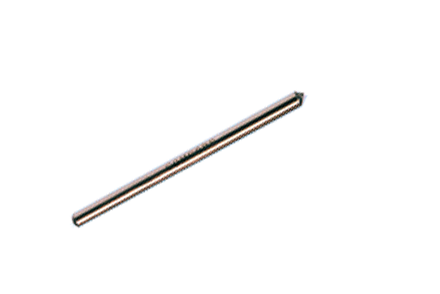### Current Position：Home / Products

 1x2(2x2) Ultra-Low PDL Narrowband SplitterProduct Features Product Applications Ultra-Low PDL Optical Communication System Low Insertion Loss Optical Testing System High Directivity Optical Fiber Sensor Stable and Reliable Optical Power Distributor
 Specifications Splitting Ratio:50:50 Parameter Unit Premium A grade Premium A grade Port Configuration 1x2 or 2x2 Bandwidth nm ±10 ±10 Insertion Loss Max dB 3.4 3.6 3.4 3.6 Excess Loss Typ dB 0.07 0.1 0.07 0.1 Uniformity Max dB 0.6 1.0 0.6 1.0 PDL Max dB 0.03 0.05 0.06 0.08 Return Loss* Min dB 55 50 55 50 Operating Power Max W 5 Operating Temperature °C -40 to +85 Storage Temperature °C -50 to +85 Package Type mm S6=Ø3x54: for bare fiber S8=Ø3x70: for 0.9mm loose tube M1=9x16x90: for 0.9mm loose tube or 2mm cable or 3mm cable

*Test at central wavelength only. There would be an unused termination port around 20cm for 1x2 version.

 Splitting Ratio & Insertion Loss Conversion Table Splitting Ratio Maximum Insertion Loss (dB) Premium A grade Output Port 1 Output Port 2 Output Port 1 Output Port 2 50:50 3.4 3.4 3.6 3.6 60:40 2.5 4.4 2.8 4.8 70:30 1.8 5.6 2.0 6.1 80:20 1.2 7.5 1.3 8.0 90:10 0.6 10.8 0.8 12 95:5 0.4 14.6 0.5 18.4 96:4 0.3 16.0 0.4 19.0 97:3 0.3 17.5 0.4 19.5 98:2 0.3 19.0 0.3 20.0 99:1 0.2 21.5 0.3 22.0
 Ordering Information L N S Wavelength 1=1625nm 2=1590nm 3=1570nm 4=1550nm 5=1480nm 6=1475nm 7=1310nm 8=1064nm 9=980nm A=850nm L=780nm P=2000nm S=Specify Structure 1=1x2 2=2x2 Splitting Ratio 99=99:1 98=98:2 97=97:3 96=96:4 95=95:5 90=90:10 80=80:20 70=70:30 60=60:40 50=50:50 ...... Grade P=Premium A=A grade Package 5=S6 7=S8 D=M1 Fiber Type 1=G652 or Equivalent 5=980-20 6=SM1060 7=SM1060 FLEX 8=980-16 9=SM780 L=Large mode area fiber Pigtail S=250μm bare fiber M=0.9mm loose tube L=3mm cable R=2mm cable Fiber Length 0=0.5m 1=0.75m 2=1.0m 3=1.5m 4=2.0m S=Specify Connector 0=None 1=FC/PC 2=FC/SPC 3=FC/APC 4=SC/SPC 5=SC/APC 6=ST 7=FC/UPC 8=SC/UPC 9=MU A=LC/PC B=SC/PC C=LC/UPC D=LC/APC

Note:1. Central wavelength can be customized for different applications.
2. All specifications are before connectors and are subject to change without notice.
3. All data are measured at central wavelength at room temperature.